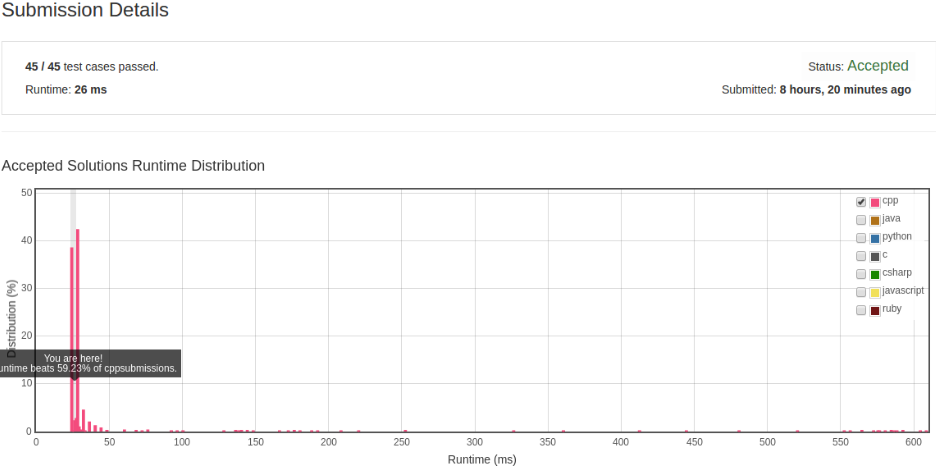# 凌云的博客

## LeetCode 算法题 11. Container With Most Water

### 题目

Given n non-negative integers a1, a2, ..., an, where each represents a point at coordinate (i, ai). n vertical lines are drawn such that the two endpoints of line i is at (i, ai) and (i, 0). Find two lines, which together with x-axis forms a container, such that the container contains the most water.

Note: You may not slant the container.

### 解法

``````class Solution {
public:
int maxArea(vector<int>& height) {
const int size = height.size();
int maxArea = 0;
for (int i = 0, j = size - 1; i != j; ) {
if (height[i] >= height[j]) {
maxArea = max(maxArea, (j - i) * (height[j]));
--j;
} else {
maxArea = max(maxArea, (j - i) * (height[i]));
++i;
}
}

return maxArea;
}
};
````````````    1 2 3 4 5 6
1 x
2 x x
3 x x x
4 x x x x
5 x x x x x
6 x x x x x x
``````

``````    1 2 3 4 5 6
1 x - - - - o
2 x x
3 x x x
4 x x x x
5 x x x x x
6 x x x x x x
``````

``````    1 2 3 4 5 6
1 x ------- o
2 x x       o
3 x x x     |
4 x x x x   |
5 x x x x x |
6 x x x x x x
``````